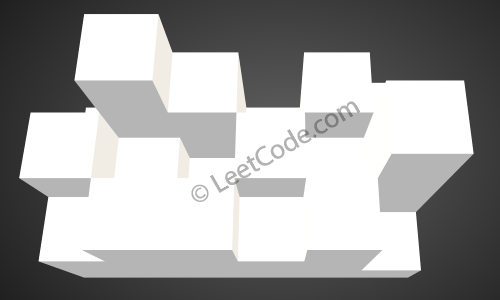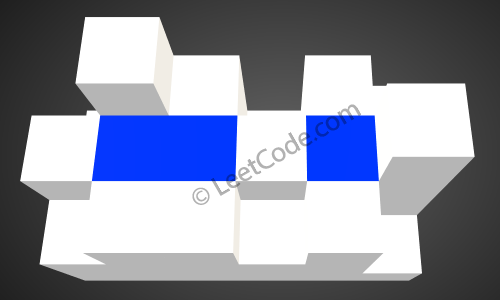## 407. Trapping Rain Water II

Given an `m x n` matrix of positive integers representing the height of each unit cell in a 2D elevation map, compute the volume of water it is able to trap after raining.

Example:

```Given the following 3x6 height map:
[
[1,4,3,1,3,2],
[3,2,1,3,2,4],
[2,3,3,2,3,1]
]

Return 4.
```The above image represents the elevation map `[[1,4,3,1,3,2],[3,2,1,3,2,4],[2,3,3,2,3,1]]` before the rain.After the rain, water is trapped between the blocks. The total volume of water trapped is 4.

Constraints:

• `1 <= m, n <= 110`
• `0 <= heightMap[i][j] <= 20000`

## Rust Solution

``````struct Solution;

use std::cmp::Reverse;
use std::collections::BinaryHeap;

impl Solution {
fn trap_rain_water(height_map: Vec<Vec<i32>>) -> i32 {
let n = height_map.len();
let m = height_map.len();
let mut queue: BinaryHeap<(Reverse<i32>, usize, usize)> = BinaryHeap::new();
let mut visited = vec![vec![false; m]; n];
for i in 0..n {
for j in 0..m {
if i == 0 || j == 0 || i == n - 1 || j == m - 1 {
visited[i][j] = true;
queue.push((Reverse(height_map[i][j]), i, j));
}
}
}
let mut res = 0;
while let Some((Reverse(h), i, j)) = queue.pop() {
if i > 0 && !visited[i - 1][j] {
let ii = i - 1;
visited[ii][j] = true;
if h > height_map[ii][j] {
res += h - height_map[ii][j];
queue.push((Reverse(h), ii, j));
} else {
queue.push((Reverse(height_map[ii][j]), ii, j));
}
}
if j > 0 && !visited[i][j - 1] {
let jj = j - 1;
visited[i][jj] = true;
if h > height_map[i][jj] {
res += h - height_map[i][jj];
queue.push((Reverse(h), i, jj));
} else {
queue.push((Reverse(height_map[i][jj]), i, jj));
}
}
if i + 1 < n && !visited[i + 1][j] {
let ii = i + 1;
visited[ii][j] = true;
if h > height_map[ii][j] {
res += h - height_map[ii][j];
queue.push((Reverse(h), ii, j));
} else {
queue.push((Reverse(height_map[ii][j]), ii, j));
}
}
if j + 1 < m && !visited[i][j + 1] {
let jj = j + 1;
visited[i][jj] = true;
if h > height_map[i][jj] {
res += h - height_map[i][jj];
queue.push((Reverse(h), i, jj));
} else {
queue.push((Reverse(height_map[i][jj]), i, jj));
}
}
}
res
}
}

#[test]
fn test() {
let height_map = vec_vec_i32![[1, 4, 3, 1, 3, 2], [3, 2, 1, 3, 2, 4], [2, 3, 3, 2, 3, 1]];
let res = 4;
assert_eq!(Solution::trap_rain_water(height_map), res);
}
``````

Having problems with this solution? Click here to submit an issue on github.